# Should DSP Undergraduate Students Study z-Transform Regions of Convergence?

Not long ago I presented my 3-day DSP class to a group of engineers at Tektronix Inc. in Beaverton Oregon . After I finished covering my material on IIR filters' z-plane pole locations and filter stability, one of the Tektronix engineers asked a question similar to:

"I noticed that you didn't discuss z-plane regions of
convergence here. In my undergraduate DSP class we
spent a lot of classroom and homework time on the
topic of z-plane regions of convergence. Was all that
effort a waste of time?"

I answered with something like: "In my experience, studying z-plane regions of convergence is useful when you're solving homework problems but you'll never need it in your real-world DSP work."

This blog points out why studying z-plane regions of convergence is one of my complaints about how DSP is taught in today's universities. If this topic interests you, please read on.

## Why Study the z-Transform

As you know, in practice, studying the z-transform of a linear time-invariant (LTI) digital system's time-domain impulse response is super useful. That transform enables us to understand a system's frequency magnitude and phase responses as well as determining the stability of a digital system.

If you're somewhat new to DSP, or you've forgotten the many ways the z-transform is used in DSP, have a look at the z-transform video tutorial at Reference .

## The Problem With Formal z-Transform Instruction

From what I've seen in college DSP textbooks ([3-5]) and online videos of college-level instruction regarding the z-transform, classroom lectures typically begin with an elaborate and elegant mathematical theory of the z-transform of infinite-length time sequences [6-11]. That classroom discussion involves material similar to this blog's Appendix A.

The professors spend a fair amount of classroom time deriving the z-transform of various infinite-length sequences, drawing the transforms' poles on the z-plane, and discussing for what values of z the transforms exist (i.e., the z-plane region of convergence where a z-transform converges to values less than infinity). At the end of class the professor may well assign the students three or four z-transform regions of converge homework problems.

The problem I have with the above classroom activity is that when using the z-transform in the real world of implementing practical stable DSP systems, with the possible exception of digital oscillators, we never work with infinite-length sequences. So the classroom discussion and homework problems regarding the z-transform of infinite-length sequences have essentially no application in practice.

Know this: for all stable LTI systems' h(n) impulse responses you will encounter in practice, the h(n) z-domain regions of convergence will be the entire z-plane (except at z = 0 and possibly z = ∞) which, of course, contains the z-plane's unit circle . Thus the discrete-time Fourier transform (DTFT) and the discrete Fourier transform (DFT) exist for all stable h(n) impulse response sequences! And that fact is why many DSP textbooks do not bother to discuss the regions of convergence of the z-transform of infinite-length sequences.

Having a limited amount of classroom lecture time, instead of professors discussing the z-transform of infinite-length sequences (sequences which do not exist in the physical world) perhaps class time would be better spent discussing more practical issues—like fractional binary numbers or how to read a commercial A/D converter's data sheet.

## Digital Oscillators

There is one kind of pseudo-infinite-length sequence you might encounter in practice. That would be when you're implementing a digital oscillator.

As shown in Appendix B, an oscillator's causal xCOS(n) cosine output sequence does have a z-transform with poles on the z-plane's unit circle, but the transform's region of convergence lies outside the unit circle. Thus, strictly speaking, neither the DTFT nor the DFT of xCOS(n) exist. However, none of the mathematical mumbo jumbo in Appendix B prevents us from building perfectly workable digital oscillators!

## Conclusion

I don't believe that 'z-transform regions of convergence of infinite-length sequences' is an appropriate topic of study for beginners in the field of DSP.

Now before some of my readers demand that I be dragged out of my office and water boarded, I will admit that the 'z-transform regions of convergence of infinite-length sequences' may well be of interest in the mathematical field of Complex Analysis (traditionally known as "the theory of functions of a complex variable") .## Appendix A: A Quick Review of z-Plane Region of Convergence

When introducing the topic of the z-transform to their undergraduate students, many university professors present various simple infinite-length x(n) time-domain sequences and their X(z) z-transforms to the students. The professors begin by giving the traditional definition of the z-transform written as: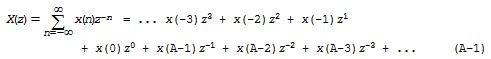where n is a time-domain index and X(z) is a continuous function of continuous complex variable z. The X($\omega$) discrete-time Fourier transform (DTFT) of x(n) is found by replacing z in Eq. (A-1) with $e^{j\omega}$ to obtain: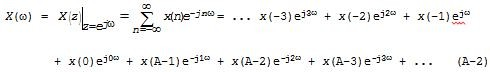Frequency variable $\omega$ is measured in radians/sample, where –$\pi$$\omega$$\pi$

Next, the main questions the professors address are, "Does X($\omega$) exist?" "That is, does the summation in Eq. (A-2) equal (converge to) a value less than infinity for all values of $\omega$?" If so, then we can say "The DTFT of x(n), X($\omega$), exists." (I.E., X($\omega$) has a physical meaning).

For X($\omega$)to exist the X(z) summation in Eq. (A-1) must converge for all values of $z = e^{j\omega}$ (the unit circle on the z-plane). So now we're faced with the crucial questions, "For what values of z in Eq. (A-1) does X(z) converge and do those values include the unit circle?" Stated in different lingo, "What is the region on the z-plane where Eq. (A-1)'s X(z) converges?" I.E., "For a given x(n) time-domain sequence what is the region of converge (ROC) of X(z)?"

If, for a given x(n), X(z) converges for values of z on the
z-plane's unit circle then x(n)'s X($\omega$) DTFT exists--and that's
what we're trying to determine here. And most important, if x(n)
is the impulse response of an LTI system and its X($\omega$) DTFT exists,
then we can compute the system's frequency response by
evaluating X($\omega$) over the range of –$\pi$$\omega$$\pi$.

To illustrate these ideas the professors present various infinite-length x(n) sequences, derive the equations for their X(z) transforms, and determine the X(z)s' regions of converge. I show examples of this in Figure A1, where the u(n) factor is the unit step sequence, values $a$ and $b$ are scalars, and the shaded areas on the right represent the regions of convergence. (Detailed descriptions of these examples can be found somewhere in References [3-12].)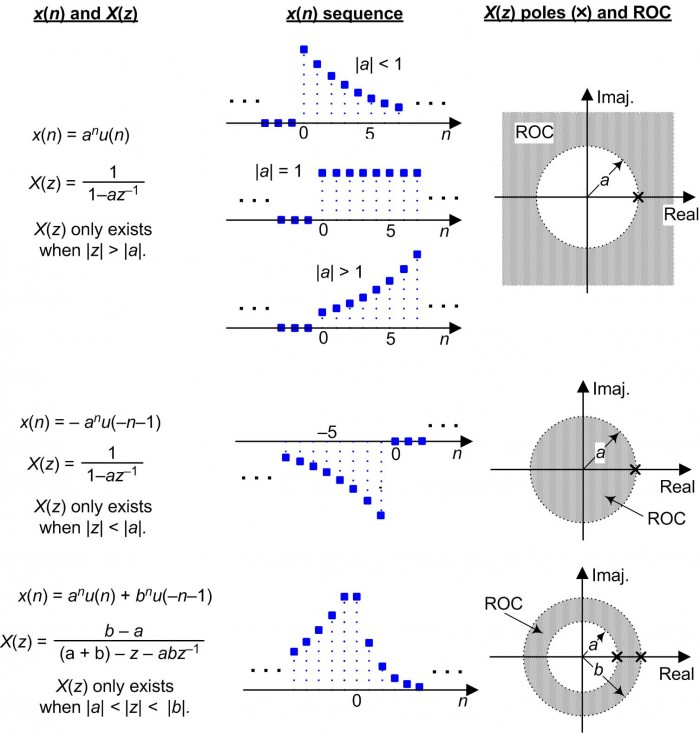Figure A1: Typical examples of infinite-length x(n) sequences,
their X(z) z-transforms, and z-plane ROCs.

## Appendix B: The z-Transform and ROC of a Causal Cosine Sequence

The z-transform of a causal xcos(n) cosine sequence (where the u(n) factor is the unit step sequence),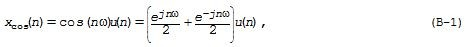is derived as follows: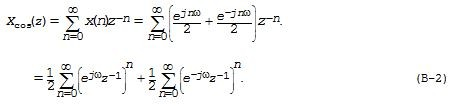In Eq. (B-2) the two summations of geometric series summations can be written in closed form as: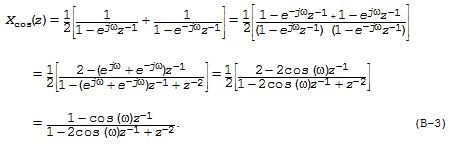The region of convergence (ROC) of Xcos(z) is found by inspecting the summations in Eq. (B-2). For Xcos(z) to converge (be less than infinity) the terms in Eq. (B-2)'s parentheses must be less than one. That is: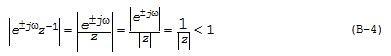Eq. (B-4) is satisfied when |z| > 1. This means that Xcos(z)'s z-plane ROC is where |z| is greater than one. We illustrate that situation in Figure B1.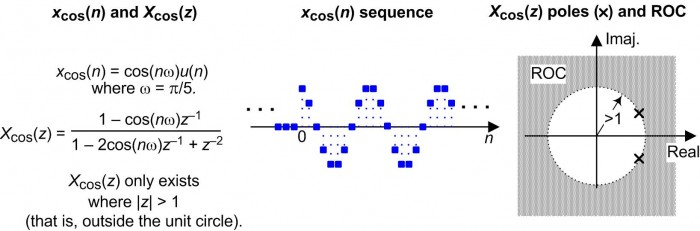Figure B1: Infinite-length xCOS(n) cosine sequence,
its XCOS(z) z-transform, and z-plane ROC.

As described on pages 54 and 97 of Reference , an infinite-length xCOS(n) cosine sequence is a very special sequence. (For example, xCOS(n) does have a z-transform but its DTFT is not strictly the z-transform evaluated on the z-plane's unit circle!) What concerns us is that sequence xCOS(n) is not absolutely summable, so its XCOS($\omega$) DTFT is not less than infinity for all values of $\omega$. Thus, strictly speaking, neither the DTFT nor the DFT of xCOS(n) exist.

However, Reference  does present a mathematical justification that a continuous periodic XCOS($\omega$) DTFT can be represented using Dirac delta impulse functions as shown in Figure B2.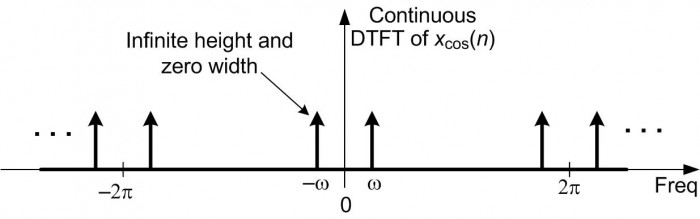Figure B2: One justifiable representation of the continuous
discrete-time Fourier transform (DTFT) of an
infinite-length time-domain cosine sequence.

References

 Lyons DSP class: http://www.besserassociates.com/Courses/Course-Description/CTID/27

 S. Mitra, Digital Signal Processing, A computer-Based Approach,
McGraw-Hill, New York, New York, 2011, pp. 283-288.

 J. Proakis and D. Manolakis, Digital Signal Processing-Principles,
Algorithms, and Applications, 3rd Ed., Prentice Hall, Upper Saddle
River, New Jersey, 1996, pp. 151-160.

 A. Oppenheim, R. Schafer, and J. Buck, Discrete-Time Signal
Processing, 2nd Ed., Prentice Hall, Englewood Cliffs, New Jersey,
1999, pp. 96-110.

 M. Beck, G. Marchesi, D. Pixton, and L. Sabalka,
"A First Course in Complex Analysis", an online book:
http://math.sfsu.edu/beck/papers/complex.pdf

[ - ]
Comment by October 2, 2016Hello MASiddiqui.
Your interesting words: "... my love for math and its application (dsp) is growing stronger each passing day." reminds me of a quote in the Preface of my brother's favorite DSP textbook. The quote is:

You see I went on with this research just the way it led me. This is the only way I ever heard of research going. I asked a question, devised some method of getting an answer, and gota fresh question. Was this possible, or that possible? You cannot imagine what this means to an investigator, what an intellectual passion grows upon him. You cannot imagine the strange colourless delight of these intellectual desires (Dr. Moreauinfamous physician and vivisectionist from H.G. Wells "Island of Dr. Moreau", 1896).

Happily MASiddiqui, in your DSP studies you are experiencing that "strange colourless delight." (I interpret that mysterious adjective "colourless" to mean "difficult to describe.")
[ - ]
Comment by September 14, 2016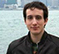In my opinion, Region of convergence or any mathematical properties of the infinite series does not belong in a DSP course. This should be taught BEFORE going into the DSP stuff, in a course of complex analysis or something like that. The Z-transform and series are just a mathematical tool to help model your real world problem. In the DSP course it should be more important to understand which of all the cases of time series happen in practice (For example, region of convergence including unitary circle and zero but not going to infinity would be an anticausal impulse response which never appears in a real system), and more of the actual use of this to do something useful.
[ - ]
Comment by September 19, 2016Anticausal systems DO exist if you include postprocessing algorithms. You can design a system that "looks into the future" of the current moment but gets the future values from already-captured data. Z-transforms are useful for this because this can only be done in a computer.
[ - ]
Comment by September 15, 2016It is possible for two or more sequences to have the exact same z-transform expression. It is the regions of convergence that serve to distinguish these sequences from one another. Without specifying the ROC you will have ambiguity as to the sequence of which you are working. While it may be the case that in practice you encounter common results that you disregard, in a class teaching signals and systems, transformations, DSP... you would be doing the students a huge disservice by not covering ROCs of the z-transform.
[ - ]
Comment by September 17, 2016Hello jrg, thanks for your thoughts.
The topic of the first two sentences in your comment are shown as the first two examples of my Figure A1. I believe those examples are of mild academic interest but of essentially no practical value when working with real-world signal sequences. jrg, I'm still in "the learning phase" with regard to DSP. If you have ever had to worry about z-plane ROC in your work with real-world sequences I'd be very interested in learning about that particular application of the z-transform.
[ - ]
Comment by September 17, 2016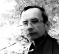Z-transform helps to understand many features of the IIR filters and properties of the discrete signal spectrum. As in the following: http://kanyevsky.kpi.ua/fpgadesign/dsp_rab2eng.html
[ - ]
Comment by September 17, 2016Hello aser,
Thanks for posting the link to Sergiyenko's interesting web page. In that web page I'm not sure it's valid to think about z^n, or e^-jw as possible inputs to a linear system. I'll have to think more about that. In any case, I agree with your comment. My Reference  shows why your comment is correct.
[ - ]
Comment by September 17, 20165 comments?, where are they? are they stolen...
[ - ]
Comment by September 17, 2016Hi Kaz,
I can see the five comments. (I'm using FireFox as my web browser.)
[ - ]
Comment by September 18, 2016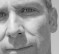Some of them needed to be approved by the moderator to be seen by everyone. You should see them now.
[ - ]
Comment by September 19, 2016Horrible! Outrageous! You were in Beaverton and didn't give me a call!

If a prof sends people home with four homework problems on ROC issues out of a semester-long class, I think that's appropriate. That much homework pretty much consists of a shot across the bow, so if at some point someone is doing advanced graduate work and actually needs to know the stuff, they've at least had warning.

What I would like to see is a justification for doing analysis of systems that involve wrapping feedback around transfer functions with unstable or metastable roots -- in those cases I always feel like there's some mathematician in pince nez glasses wagging their finger at me for violating formalism.
[ - ]
Comment by October 1, 2016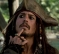Sorry to jump in the middle of big boys, but I would like to pass a comment. I see another out shoot, i.e. science broke out of philosophy because according to science philosophy does not give answers to questions. And in this topic I see similar remarks about 'ROC' that it does not play any role in real world. In calculus I was taught about roc but didn't paid attention to it and I didn't understand its implication at that time. But when i started to learn about z-transforms and saw how mathematical equations define real world phenomena, my love for math and its application (dsp) is growing stronger each passing day.
[ - ]
Comment by October 13, 2016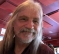i agree with both the sentiment and content of Rick's blog.

other than a homework question and maybe another on an exam, i never really ever did anything with ROC.

but, at least regarding ROC, you'll get the mathematicians and the DSP engineers agreeing about the actual math.  another, sorta similar issue, is the role of the Dirac impulse function as an actual function.  turns out that it's not precisely a function, from the mathematicians POV, even though EEs and physicists treat it as a function all of the time.

personally, i never worry about ROC nor do i worry about "the Dirac delta is a 'distribution' rather than a 'function'" issue.

To post reply to a comment, click on the 'reply' button attached to each comment. To post a new comment (not a reply to a comment) check out the 'Write a Comment' tab at the top of the comments.